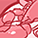# USB superspeed peripherals Forum DiscussionsLevel 2
Level 2# RAW10 to YUV

Hi,

Streaming RAW10 Format Input Data to 16/24-bit Out... - Infineon Developer Community

we understood that there is a calculation needed for changing RAW10 to YUV output format, can you please detail on how to Re-Writing the calculations.

Example 2: 16-bit output data format (CY_U3P_CSI_DF_YUV422_8_2)

Set CY_U3P_CSI_DF_YUV422_8_2 as the output format in cyu3mipicsi.c by replacing CY_U3P_CSI_DF_RAW10, to remove padded zeros.

Assume that you are streaming 1920 x 1080 resolution at 30 fps in RAW 10 format. The calculations of width in pixel, height in pixel, bits per pixel, frames per second, frame size and bit rate are as follows:

a. Width in pixel                  : 1920

b. Height in pixel                : 1080

c. Bits per pixel                  : 10

d. Frames per second       : 30

e. Frame size                      : 1920 x 1080 x 10 bits

f. Bit rate                             : 1920 x 1080 x 10 x 30 bits per second

Re-writing the above calculations:

a. Width in pixel                  : 2400

b. Height in pixel                : 540

c. Bits per pixel                  : 16

d. Frames per second       : 30

e. Frame size                      : 1200 x 1080 x 16 bits

f. Bit rate                             : 1200 x 1080 x 16 x 30 bits per secondModeratorModerator# Re: RAW10 to YUV

Hello,

If CY_U3P_CSI_DF_YUV422_8_2 is set as output the frame size will remain the same (As there is  no zero padding) which means

1920 x 1080 x 10 bits = X x Y x 16 bits , here X & Y can be defined by user provided the conditions holds true

1920 *1080 *10 = 2400 *540 *16 = 2,07,36,000

Regards,
Rashi